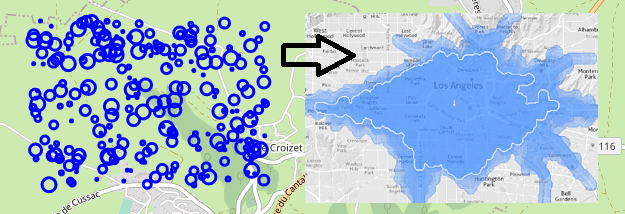# #StackBounty: #python #dataframe #interpolation #raster #folium create interpolated polygons from GPS data with value column

### Bounty: 100

I have some GPS data with a value assigned to each point (think like air quality). I can plot those points, with folium for instance, and map the value to the size of a circle, like this:

``````import pandas, numpy, folium
lat = numpy.random.uniform(45, 45.01, 250)
lon = numpy.random.uniform(3, 3.02, 250)
value = numpy.random.uniform(0,50,250)
df = pandas.DataFrame({'lat': lat, 'lon': lon, 'value': value})
mymap = folium.Map(location = [lat.mean(), lon.mean()], tiles="OpenStreetMap", zoom_start=14)
for elt in list(zip(df.lat, df.lon, df.value)):
mymap.save('mymap.html')
``````And I would like to treat this data, interpolate it and create a shapefile as the output, with interpolated polygons containing the average value and showing areas of high and low value (fake picture on the right). The limit of the polygons would auto-generated, of course, from the interpolation.

How can I achieve this? Because i have tried using HeatMap tool in folium, but it is designed to interpolate the density of points, not the value associated with each point!
I hope it’s not too complex..Thanks,
note: I use folium but i’m ok with other python libraries.

Get this bounty!!!

This site uses Akismet to reduce spam. Learn how your comment data is processed.